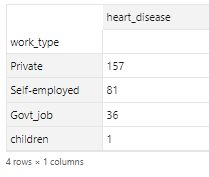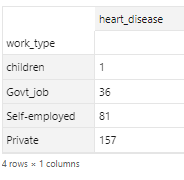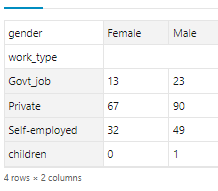# Quick EDA using Pandas Plots

In this blog, I will discuss creating plots using pandas. I will discuss the commonly used plots in Exploratory Data Analysis : scatter plot, bar plot and box plot.

Dataset — Brain Stroke Dataset from Kaggle

Resource — Pandas Documentation

Pandas plot has many arguments. The important arguments are the following:

• x
• y
• kind

— ‘line’ : line plot (default)

— ‘bar’ : vertical bar plot

— ‘barh’ : horizontal bar plot

— ‘hist’ : histogram

— ‘box’ : boxplot

— ‘kde’ : Kernel Density Estimation plot

— ‘density’ : same as ‘kde’

— ‘area’ : area plot

— ‘pie’ : pie plot

— ‘scatter’ : scatter plot (DataFrame only)

— ‘hexbin’ : hexbin plot (DataFrame only)

• ax
• subplots
• figsize — a tuple (width, height) in inches
• title — str or list (List is used for subplots)
• xticks
• yticks
• xlim
• ylim
• xlabel
• ylabel
• stacked

First, we will import the libraries and load the data

`import pandas as pddf = pd.read_csv("brain_stroke.csv")`

We will take a look at the data using head()

`df.head(n=10)`
1. Scatter Plot — It can be created using two ways
• df.plot(x = ‘col1’, y = ‘col2’, kind = ‘scatter’)
• df.plot.scatter(x = ‘col1’, y = ‘col2’)
• Other important arguments are : alpha-transparency, color-color, s-size, marker-marker type
`df.plot(x = 'age', y = 'avg_glucose_level', kind = 'scatter', alpha = 0.2, color = 'red', s=0.5, marker = "*",title = 'scatter plot between age and avg glucose level',xlabel = 'Age', ylabel = 'Avg Glucose Level')`
`df.plot.scatter(x = 'bmi', y = 'avg_glucose_level', alpha = 0.2, color = 'red', s=0.5, marker = "*",title = 'scatter plot between bmi and avg glucose level',xlabel = 'BMI', ylabel = 'Avg Glucose Level')`

2. Bar plot — It can be created using summarized data

• df.plot(x = ‘col1’, y = ‘col2’, kind = ‘bar’)
• df.plot.bar(x = ‘col1’, y = ‘col2’)
`# Summarize the data by work type. The summarized data is sorted in decreasing order by values# The index of the data frame is work_typedf_summ = df.groupby(['work_type'])['heart_disease'].agg('sum').sort_values(ascending=False)df_summ.head()`Count of Heart Disease by Work Type
`# Plot the summarized data as bar plot# The index is used as x-axis# The column is used as y-axisdf_summ.plot(kind = 'bar', color = 'blue',title = 'Heart Disease by Work Type',xlabel = 'Work Type', ylabel = 'Count',rot = 30)`

3. Horizontal Bar plot — It can be created on summarized data using

• df.plot(x = ‘col1’, y = ‘col2’, kind = ‘barh’)
• df.plot.barh(x = ‘col1’, y = ‘col2’)
`# Summarize the data by work type. The summarized data is sorted in ascending order by values# The index of the data frame is work_typedf_summ = df.groupby(['work_type'])['heart_disease'].agg('sum').sort_values(ascending=True)df_summ.head()`Count of Heart Disease by Work Type
`# Plot the summarized data as horizontal bar plot# The index is used as y-axis# The column is used as x-axisdf_summ.plot(kind = 'barh', color = 'magenta',title = 'Heart Disease by Work Type',xlabel = 'Work Type', ylabel = 'Count',rot  = 30)`

4. Stacked/Dodged Bar Plot — It can be created by summarizing the data and unstacking the summarized data

`# Summarize the data by Work Type and Gender# Unstack to get separate columns for Female and Maledf_summ = df.groupby(['work_type', 'gender'])['heart_disease'].agg('sum')df_summ = df_summ.unstack()df_summ`Heart Disease by Work Type and Gender
`# Plotting the unstacked summarized data# stacked = True stacks the barsdf_summ.plot(kind = 'bar', color = {"Male":"blue", "Female":"pink"}, stacked = True,title = 'Heart Disease by Work Type for Female and Male',xlabel = 'Work Type', ylabel = 'Count',rot  = 30)`

Dodged Bar plot

`# Plotting the unstacked summarised datadf_summ.plot(kind = 'bar', color = {"Male":"blue", "Female":"pink"}, stacked = False,title = 'Heart Disease by Work Type for Female and Male',xlabel = 'Work Type', ylabel = 'Count',rot  = 30)`

Subplots

`# Plotting the unstacked summarized datadf_summ.plot(kind = 'bar', color = {"Male":"blue", "Female":"pink"}, subplots = True,title = 'Heart Disease by Work Type for Female and Male',xlabel = 'Work Type', ylabel = 'Count',rot  = 30)`

5. Histogram — Select the column of the dataframe for histogram and plot using

• df[‘age’].plot(kind = ‘hist’, bins = 30) or
• df[‘age’].plot.hist(bins = 30)
`df['age'].plot(kind = 'hist', bins = 30, title = 'Histogram of age for bins 30')`

6. Box— Select the column of the dataframe for box plot and plot using

• df[‘age’].plot(kind = ‘box’) or
• df[‘age’].plot.box()
`df['age'].plot(kind = 'box')`

7. 100% Stacked Bar plot — It can be prepared by summarizing the data with percentage calculations

`# 100% Stacked# Summarize the data by Calculating Sum of heart disease by Work Type and Gender# Unstack to get separate columns for Female and Maledf_summ = df.groupby(['work_type', 'gender'])['heart_disease'].agg('sum')df_summ = df_summ.unstack()#Create a new column with total for male and femaledf_summ['All'] = df_summ['Female'] + df_summ['Male']# Percentage for Male and Femaledf_summ['Male_perc'] = df_summ['Male']/df_summ['All']*100df_summ['Female_perc'] = df_summ['Female']/df_summ['All']*100df_summ`
`# Plotting the unstacked summarized data# stacked = True stacks the barsdf_summ[['Male_perc', 'Female_perc']].plot(kind = 'bar', color = {"Male_perc":"blue", "Female_perc":"pink"}, stacked = True,title = 'Heart Disease % by Work Type for Female and Male',xlabel = 'Work Type', ylabel = 'Count',rot  = 0)`

Please share the blog if you like it. Please follow as it motivates me to write more.

--

--## Jyoti Kumar

I have experience in Predictive Modelling and Dashboards. I have rich working experience on various tools and software like Python, R, Tableau, Power BI and SQL